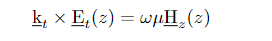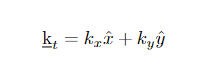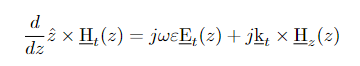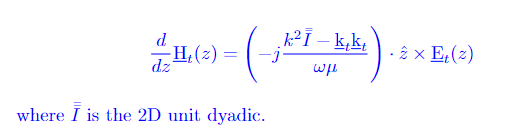# Proving an equation in a book about Periodic Structures

• A
• haji-tos

#### haji-tos

TL;DR Summary
Proving an equation in a book
Hello everyone,

I am reading some book titled: Periodic Structures: Mode-Matching Approach and Applications in Electromagnetic Engineering.
In Chapter 2, there is an equation as follows:where. Here the electric field is along the transverse x − y plane like the propagation vector kt.

Now it is said that if we substitute the equation above in the following equation by getting rid of H_z:we will obtain the following:.

When trying to prove this, I don't get the same result. It's been a long time that I am trying to prove this. Can someone please help me prove if this is true ?

Thank you very much.

The following two vector identities are very helpful: $$\vec A \times \left( \vec B \times \vec C \right) = \vec B\left(\vec A \cdot \vec C \right) - \vec C\left(\vec A \cdot \vec B \right)$$ $$\vec A \cdot \left( \vec B \times \vec C \right) =\vec B \cdot \left( \vec C \times \vec A \right)$$
Consider the given equation $$\frac d {dz} \hat z \times \vec H_t = j \omega \varepsilon \vec E_t + j \vec k_t \times \vec H_z$$ This can be written as $$\hat z \times \frac {d \vec H_t} {dz} = j \omega \varepsilon \vec E_t + j \vec k_t \times \vec H_z$$ Apply ##\hat z \times## to both sides: $$\hat z \times \left(\hat z \times \frac {d \vec H_t} {dz}\right) = j \omega \varepsilon \hat z \times \vec E_t + j \hat z \times \left(\vec k_t \times \vec H_z \right).$$ Use the first vector identity given above to show that this may be reduced to $$\frac {d \vec H_t} {dz}= -j\omega\varepsilon \hat z \times \vec E_t - j \vec k_t \left(\hat z \cdot \vec H_z \right) \,\,\,\,\,\,\,\,\, \rm Eq. 1$$

From the given equation ##\vec k_t \times \vec E_t = \omega \mu \vec H_z## we have ##\vec H_z = \frac 1 {\omega \mu} \vec k_t \times \vec E_t##. Substitute this into the last term of Eq. 1 above. Use the second vector identity to finally obtain $$\frac {d \vec H_t} {dz}= -j\omega\varepsilon \hat z \times \vec E_t + \frac j {\omega \mu} \vec k_t \left[\vec k_t \cdot \left( \hat z \times \vec E_t \right) \right] \,\,\,\,\,\,\,\,\, \rm Eq. 2$$ This can be written in the dyadic form that is given in the first post. You will need to know how ##\varepsilon## is related to ##\omega, k## and ## \mu##.

•PhDeezNutz, haji-tos and vanhees71